# Area Under a Curve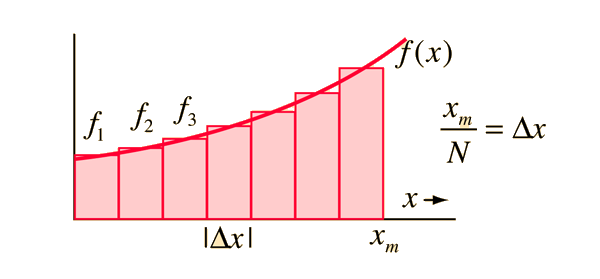Formulating the area under a curve is the first step toward developing the concept of the integral. The area under the curve formed by plotting function f(x) as a function of x can be approximated by drawing rectangles of finite width and height f equal to the value of the function at the center of the interval.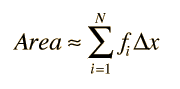If the width of the rectangles is made smaller, then the number N is larger and the approximation of the area is better.

### Show area approximation of an integral.

Index

 HyperPhysics*****HyperMath*****Calculus R Nave
Go Back

# Integral as Limit of Area

The approximation to the area under a curve can be made better by making the approximating rectangles narrower. The idea of the integral is to increase the number of rectangles N toward infinity by taking the limit as the rectangle width approaches zero.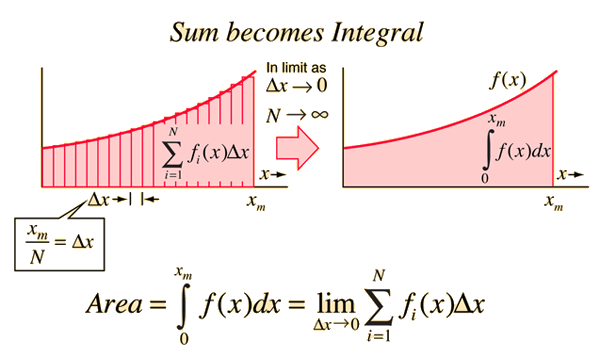While the concept of geometrical area is a convenient way to visualize an integral, the idea of integration is much more general than that. Any continuous physical variable can be "chopped up" into infinitesmal increments (differential elements) so that the sum of the product of that "width" and some function approaches an infinite sum. The integral is a powerful tool for modeling physical problems which involve continuously varying quantities.

### Show area integral examples.

Index

Integrals

 HyperPhysics*****HyperMath*****Calculus R Nave
Go Back

# Area Integral Examples

Area examples with simple geometry can reinforce the idea of the integral as the area under a curve. For a function which is just a constant a, then the area formed by the function is just a rectangle.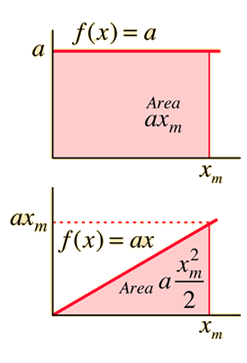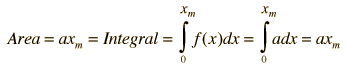The general conclusion here is that the integral of a constant is just that constant times the variable of integration x. For a function f(x) = ax, the area is a triangle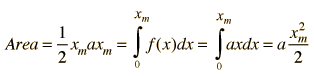The progression is to the general form for the integral of a polynomial in x:Index

 HyperPhysics*****HyperMath*****Calculus R Nave
Go Back

# Area Integral Approximations

The area under any continuous curve can be approximated by drawing a number of rectangles. The integral is the limit for an infinite number of rectangles.### Show the integral which is the limiting case.

Index

 HyperPhysics*****HyperMath*****Calculus R Nave
Go Back

# Area Integral Example

Integrals are useful for finding the area under curves which can be approximated by geometrical methods.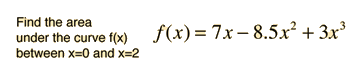This is a polynomial type integral which can be found as the sum of the integrals of its parts.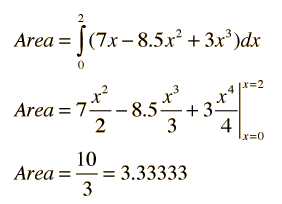### Application to the average of a function

Index

 HyperPhysics*****HyperMath*****Calculus R Nave
Go Back